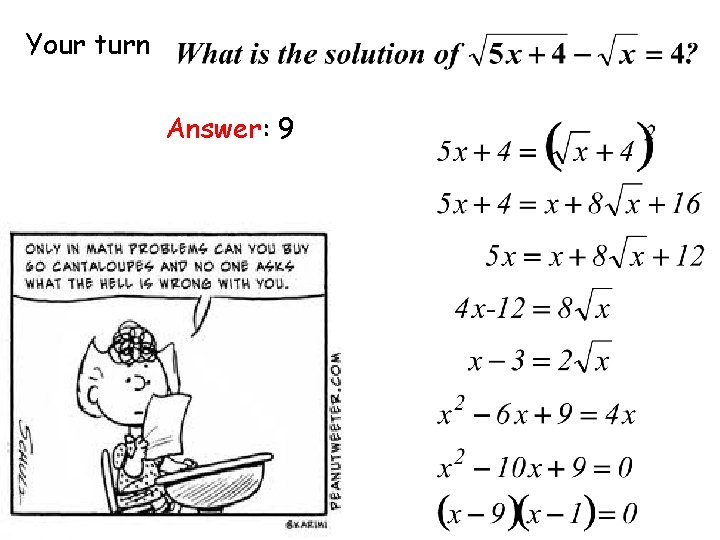# Solving Square Root and Other Radical Equations n

• Slides: 11Solving Square Root and Other Radical Equations n r a e l l you’l What Solve square root and other radical equations. y r a l u b a Voc Radical Equation: Any equation that includes a variable inside a radicand (or with a fractional exponent) Square root equation: To solve radical equations, isolate the radical on one side of the equal sign, and raise both sides of the equation to the necessary power to remove the radical sign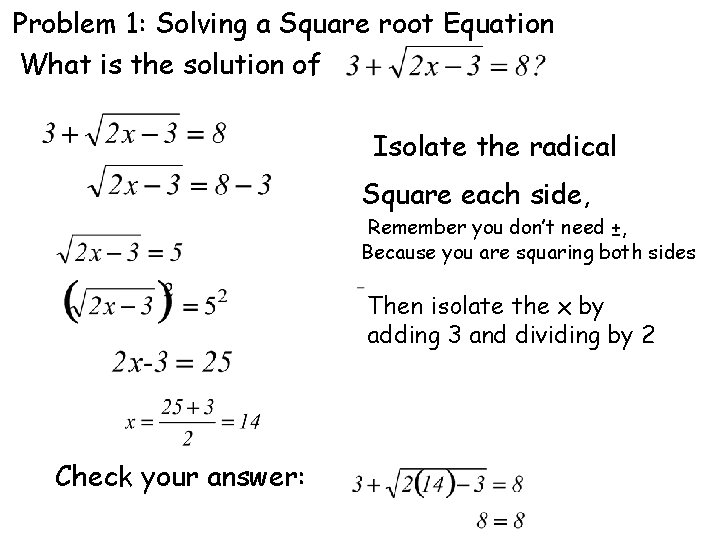Problem 1: Solving a Square root Equation What is the solution of Isolate the radical Square each side, Remember you don’t need ±, Because you are squaring both sides Then isolate the x by adding 3 and dividing by 2 Check your answer: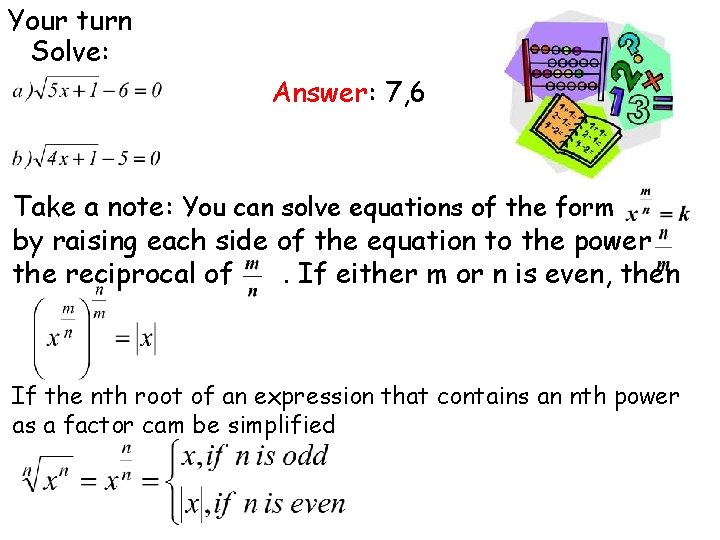Your turn Solve: Answer: 7, 6 Take a note: You can solve equations of the form by raising each side of the equation to the power the reciprocal of. If either m or n is even, then If the nth root of an expression that contains an nth power as a factor cam be simplified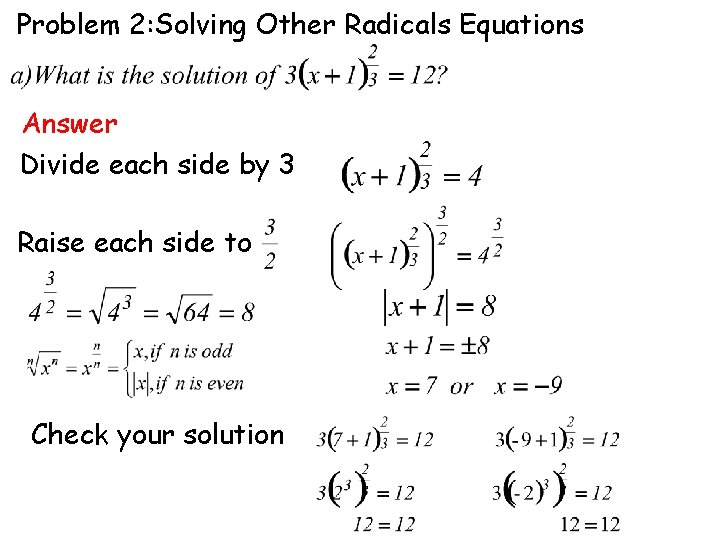Problem 2: Solving Other Radicals Equations Answer Divide each side by 3 Raise each side to Check your solution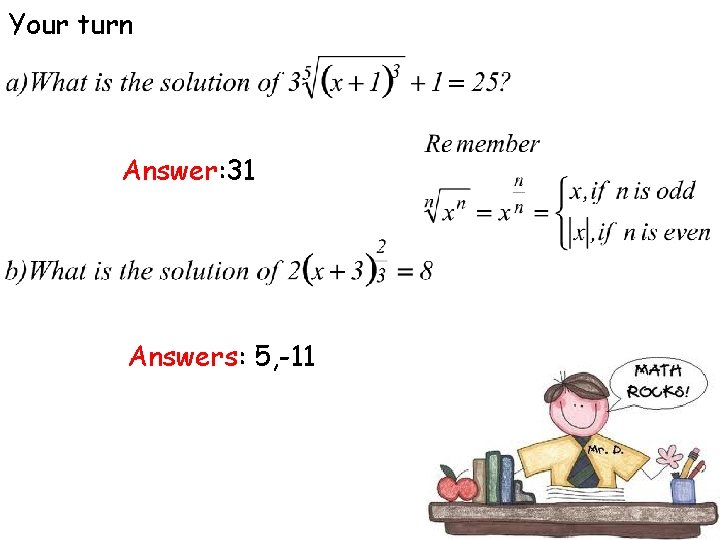Your turn again For meteor Crater in Arizona, the formula Relates the diameter d of the rim in meters to the volume V in cubic meters. What is the volume of meteor crater? All values are approximate. Answer 1. 2 kmWhen you raise each side of an equation to a power, it is possible to introduce extraneous solutions in the original equation, it becomes very important to check all solutions in the original equation. A Correct solution will give you a true statement. An extraneous solution will give you a false statement. Problem 3: Checking for extraneous solutions Answer Isolate the radical Square each side Simplify Combine like terms Factor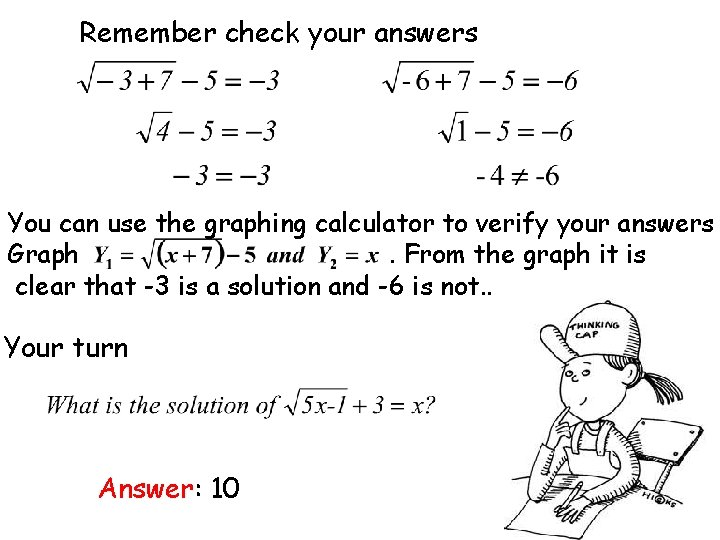Remember check your answers You can use the graphing calculator to verify your answers Graph. From the graph it is clear that -3 is a solution and -6 is not. . Your turn Answer: 10Problem 4: Solving an Equation with two Radicals Isolate the more complicated radical Square each side Solve, combine like terms Square each side Check